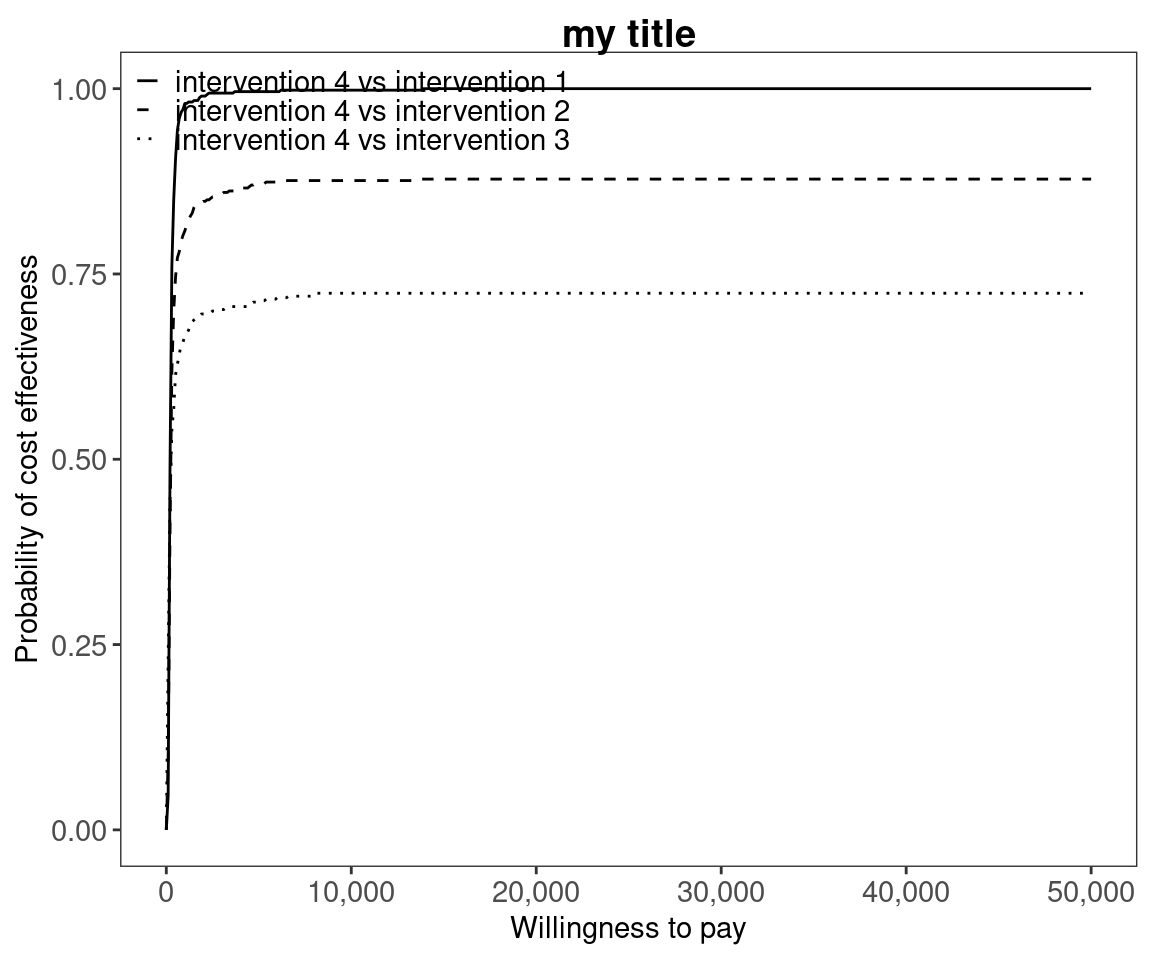Cost-effectiveness acceptability curve plots

Introduction

The intention of this vignette is to show how to plot different styles of cost-effectiveness acceptability curves using the BCEA package.

Two interventions only

This is the simplest case, usually an alternative intervention ($$i=1$$) versus status-quo ($$i=0$$).

The plot show the probability that the alternative intervention is cost-effective for each willingness to pay, $$k$$,

$p(NB_1 \geq NB_0 | k) \mbox{ where } NB_i = ke - c$

Using the set of $$N$$ posterior samples, this is approximated by

$\frac{1}{N} \sum_j^N \mathbb{I} (k \Delta e^j - \Delta c^j)$

R code

To calculate these in BCEA we use the bcea() function.

data("Vaccine")

he <- bcea(e, c)
#> No reference selected. Defaulting to first intervention.
# str(he)

ceac.plot(he)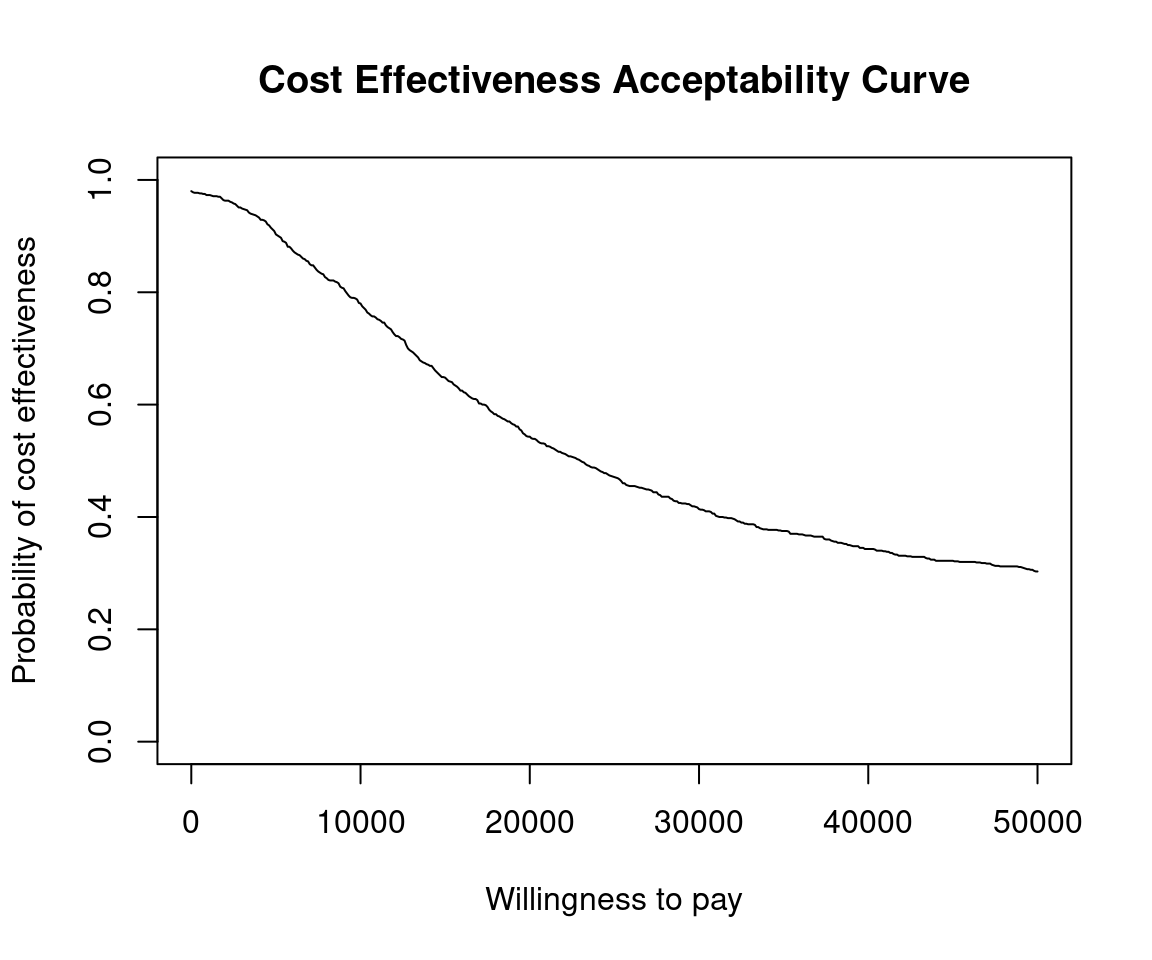The plot defaults to base R plotting. Type of plot can be set explicitly using the graph argument.

ceac.plot(he, graph = "base")ceac.plot(he, graph = "ggplot2")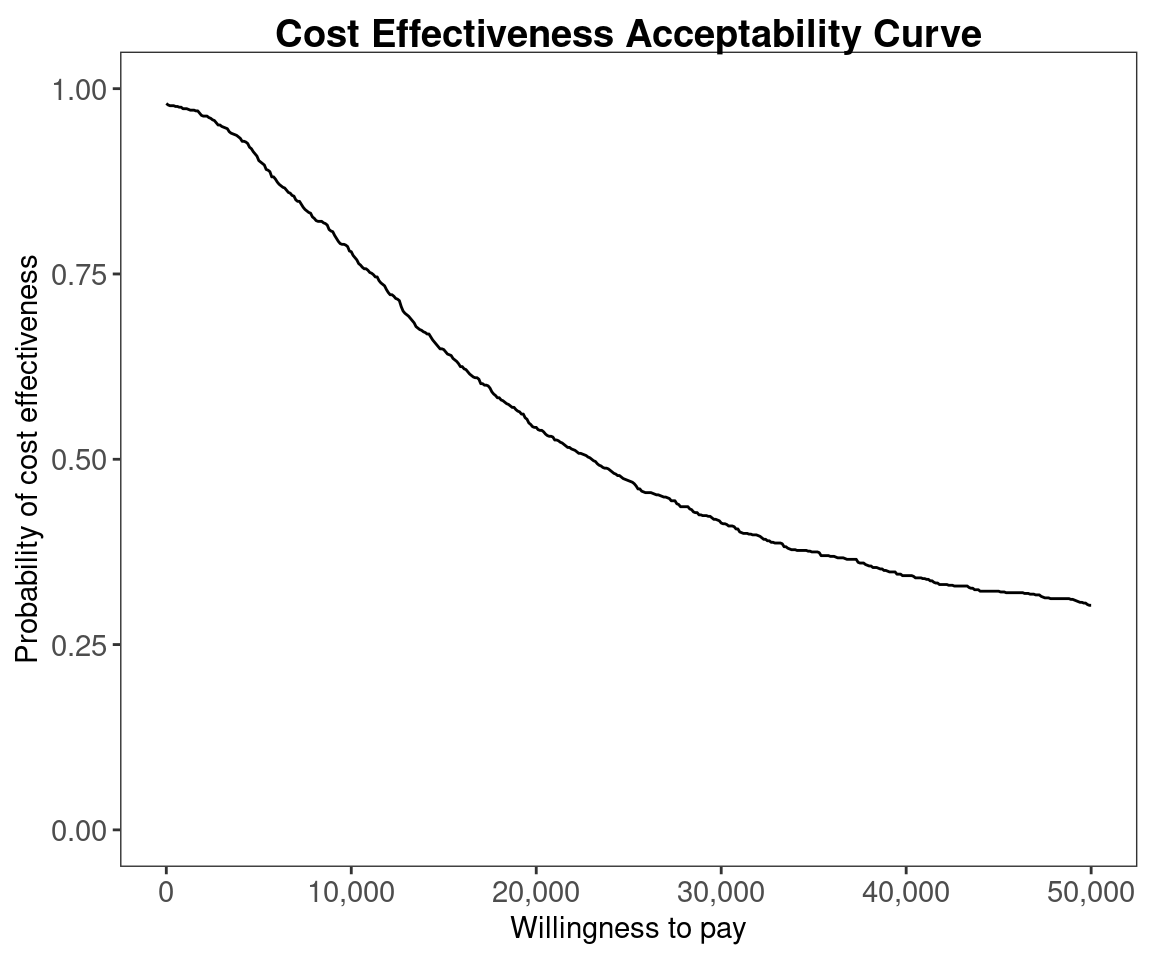# ceac.plot(he, graph = "plotly")

Other plotting arguments can be specified such as title, line colours and theme.

ceac.plot(he,
graph = "ggplot2",
title = "my title",
line = list(colors = "green"),
theme = theme_dark())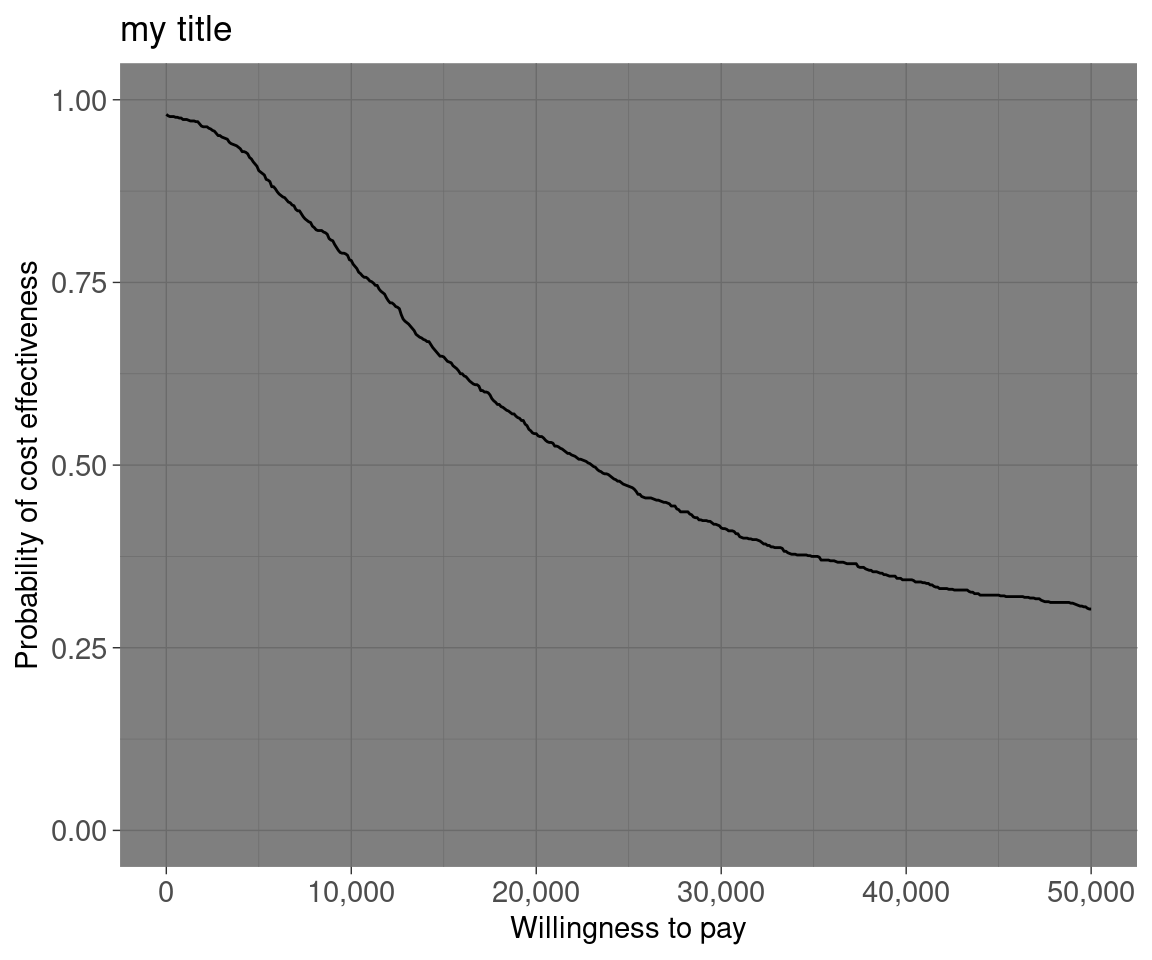Multiple interventions

This situation is when there are more than two interventions to consider. Incremental values can be obtained either always against a fixed reference intervention, such as status-quo, or for all pair-wise comparisons.

Against a fixed reference intervention

Without loss of generality, if we assume that we are interested in intervention $$i=1$$, then we wish to calculate

$p(NB_1 \geq NB_s | k) \;\; \exists \; s \in S$

Using the set of $$N$$ posterior samples, this is approximated by

$\frac{1}{N} \sum_j^N \mathbb{I} (k \Delta e_{1,s}^j - \Delta c_{1,s}^j)$

R code

This is the default plot for ceac.plot() so we simply follow the same steps as above with the new data set.

data("Smoking")

he <- bcea(e, c, ref = 4)
# str(he)
ceac.plot(he)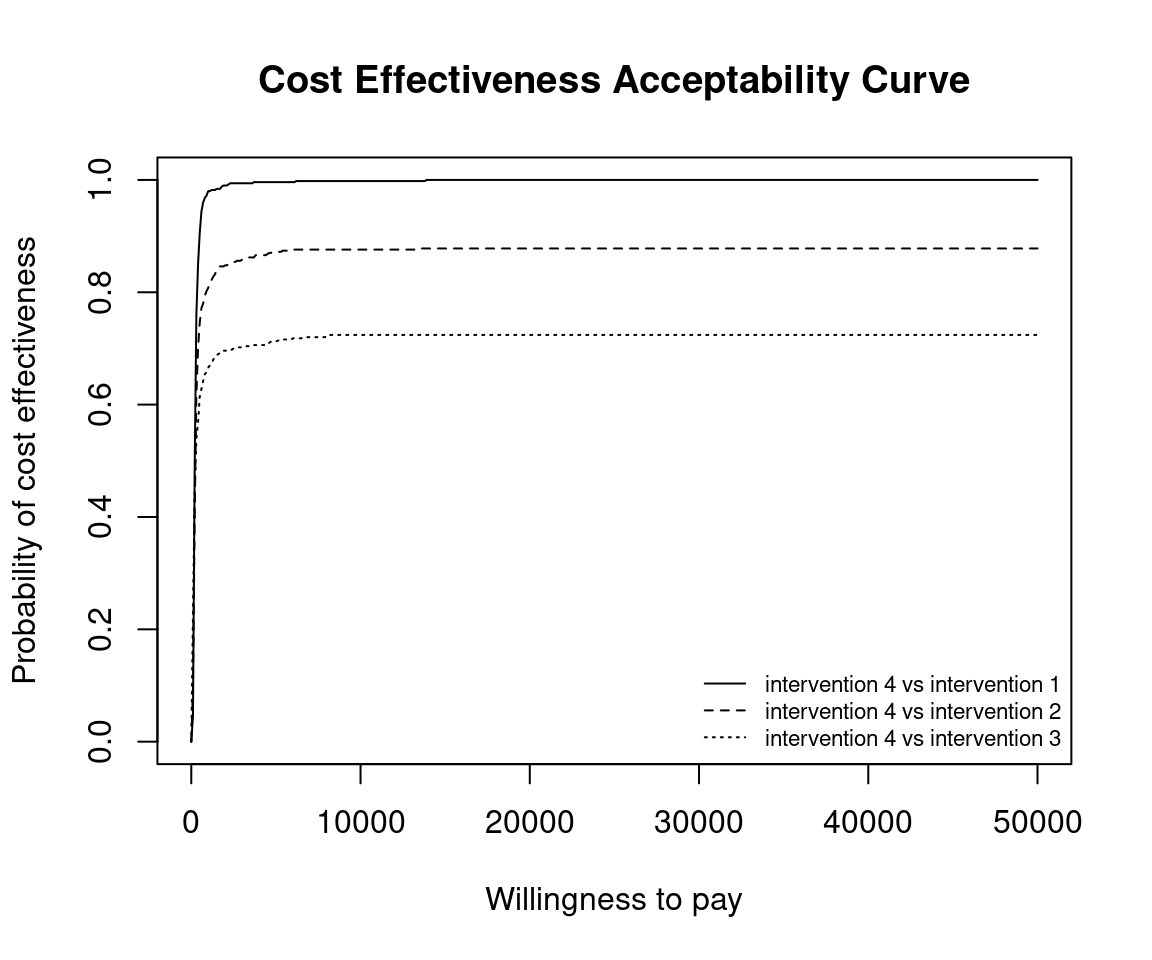ceac.plot(he,
graph = "base",
title = "my title",
line = list(colors = "green"))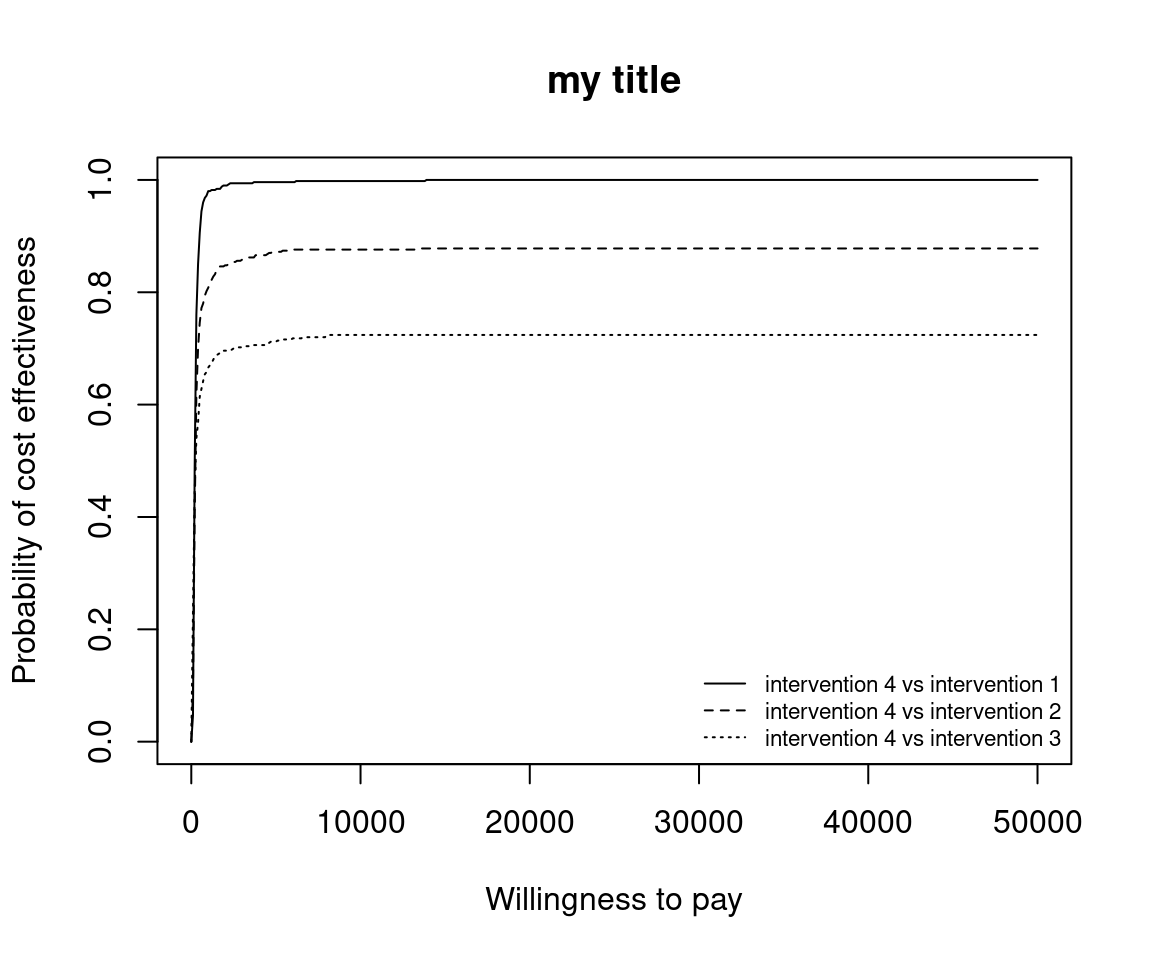ceac.plot(he,
graph = "ggplot2",
title = "my title",
line = list(colors = "green"))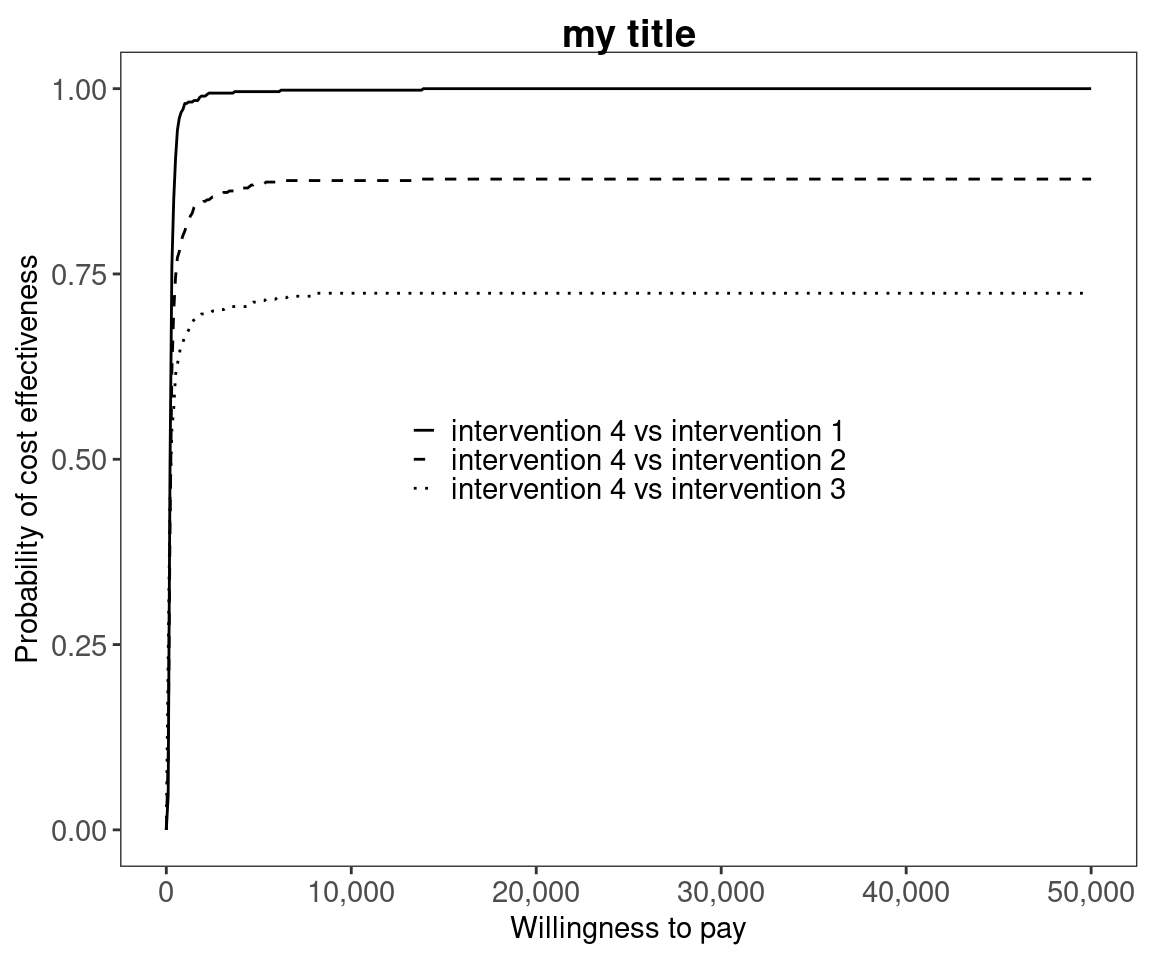Reposition legend.

ceac.plot(he, pos = FALSE) # bottom rightceac.plot(he, pos = c(0, 0))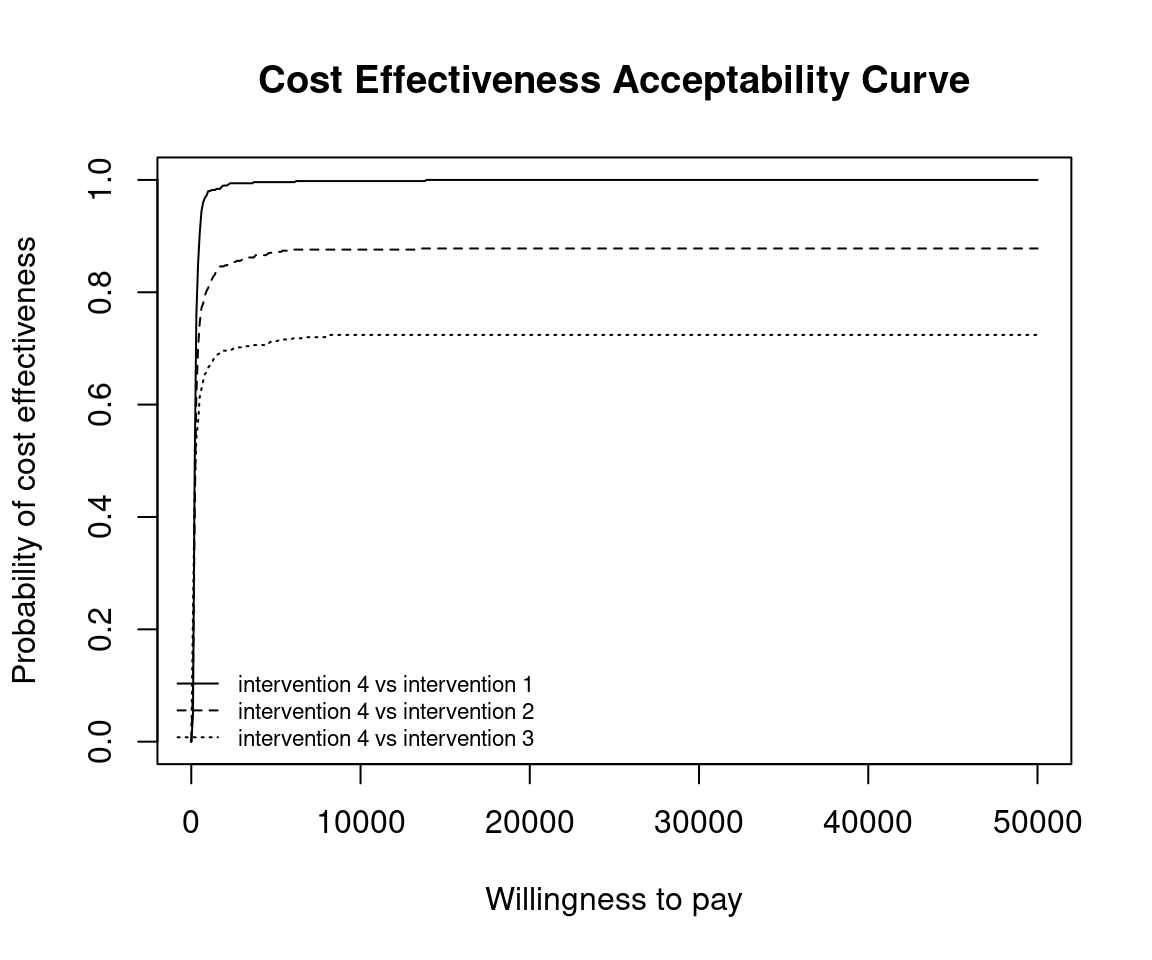ceac.plot(he, pos = c(0, 1))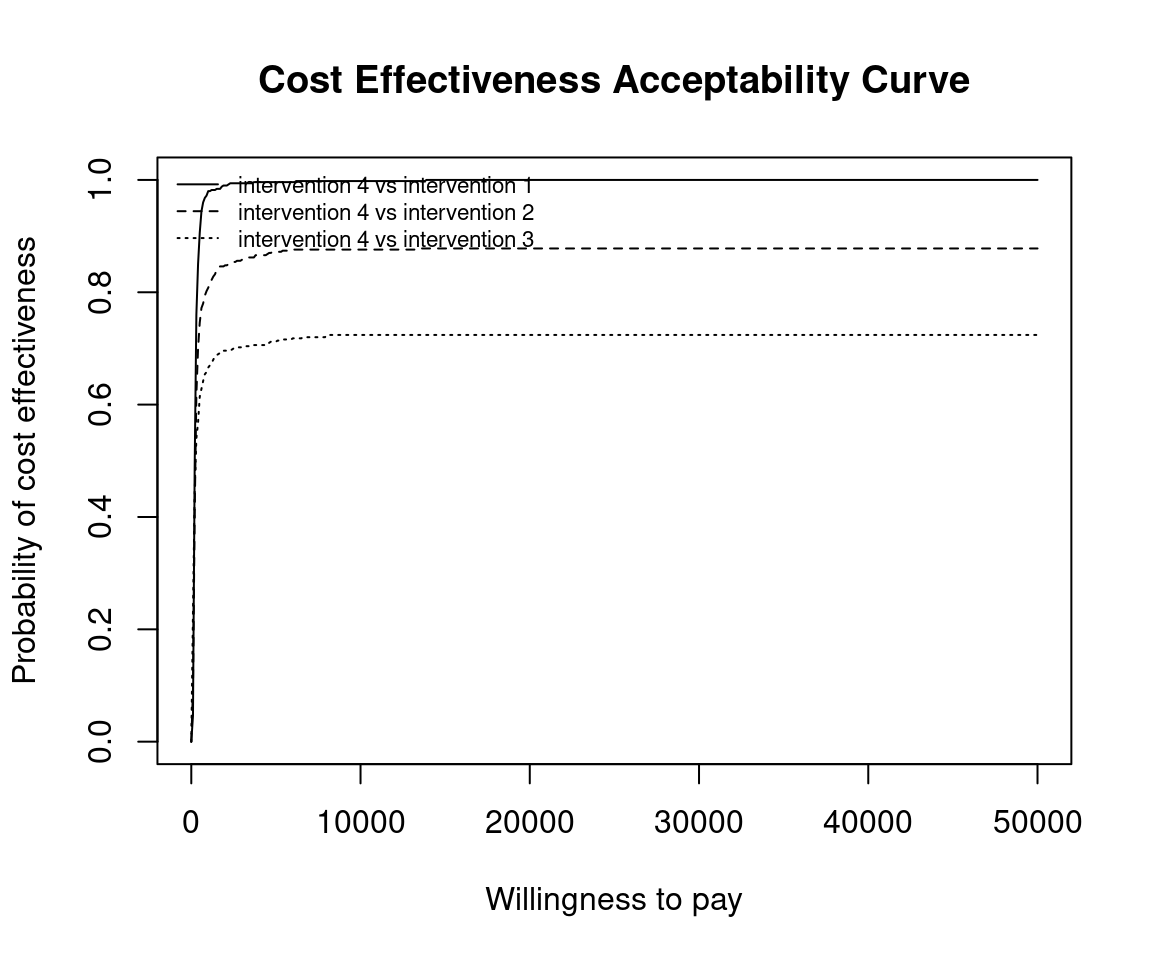ceac.plot(he, pos = c(1, 0))ceac.plot(he, pos = c(1, 1))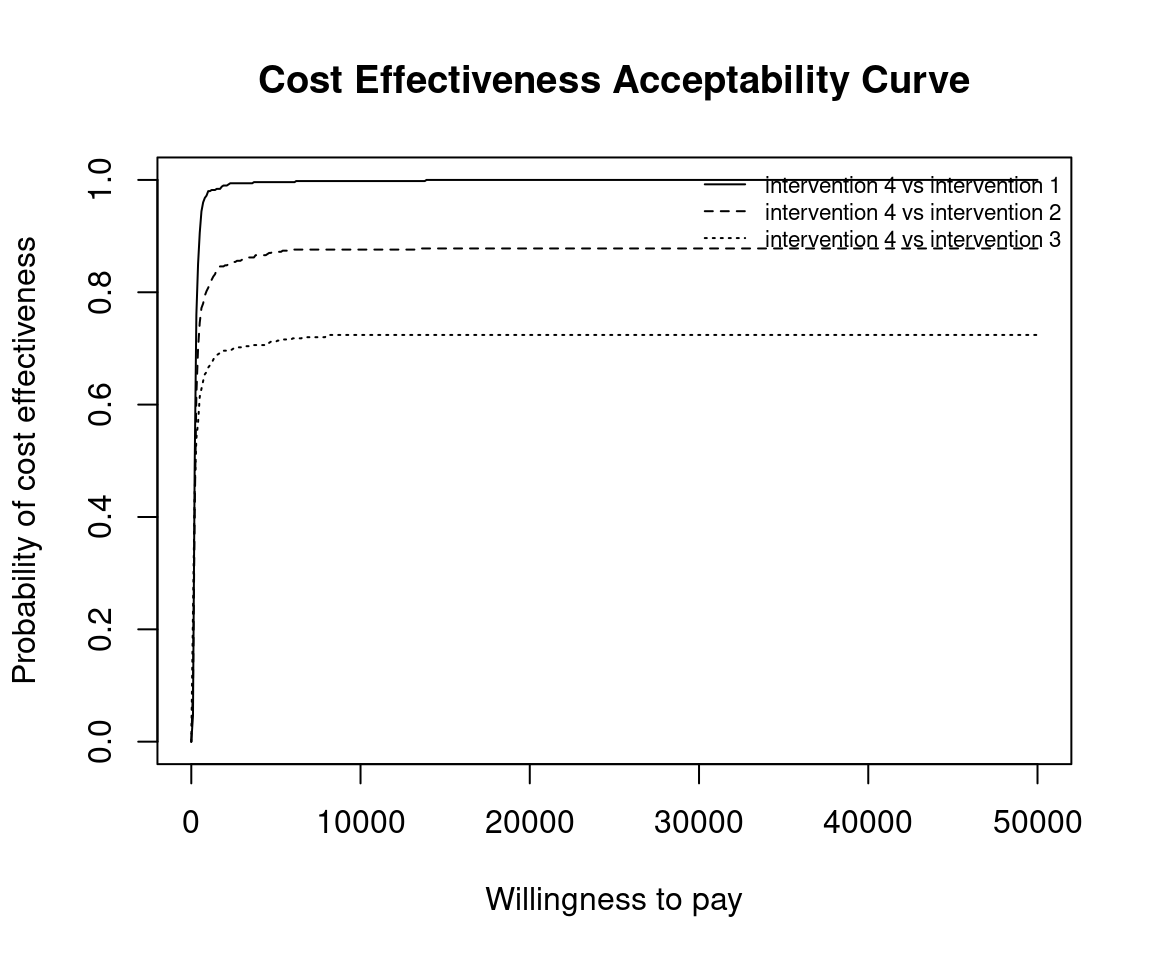ceac.plot(he, graph = "ggplot2", pos = c(0, 0))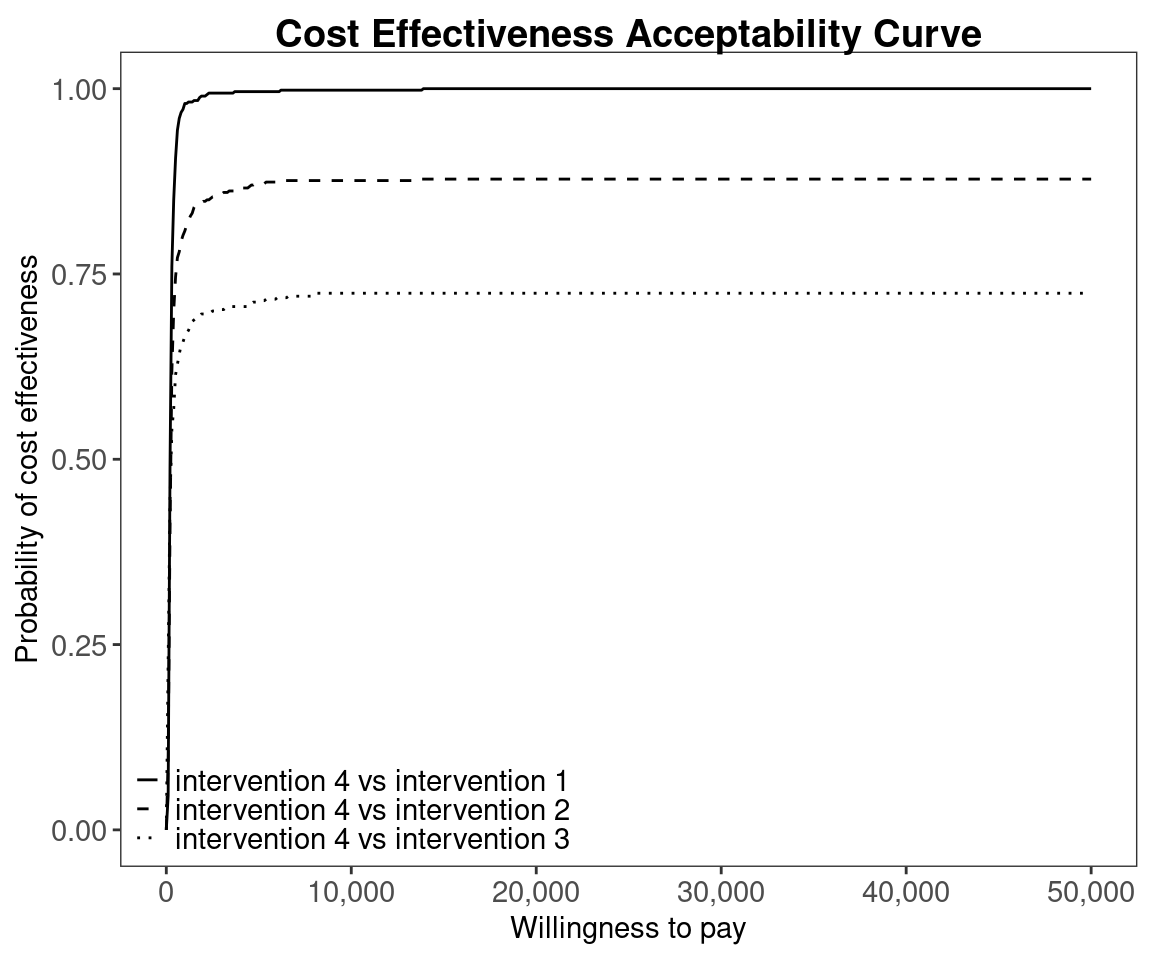ceac.plot(he, graph = "ggplot2", pos = c(0, 1))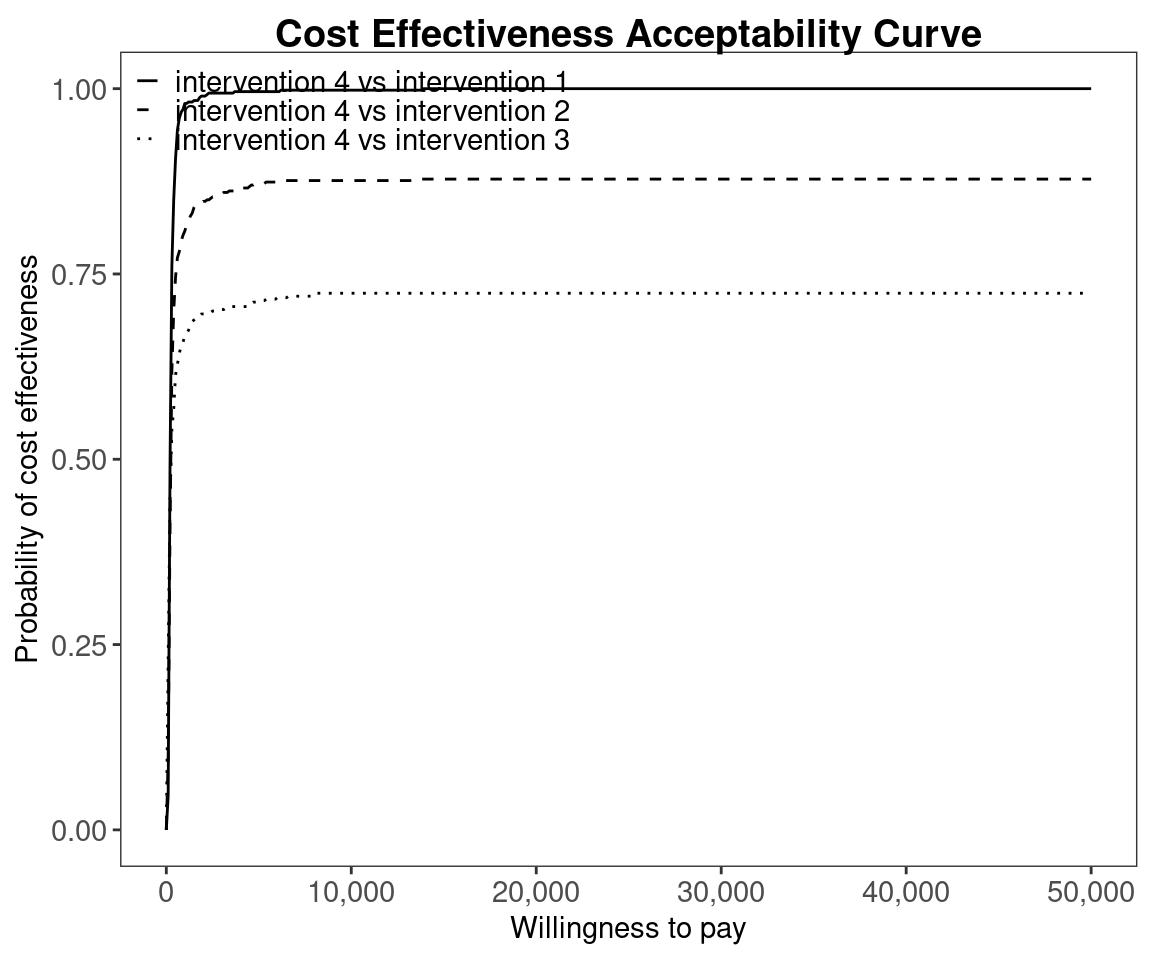ceac.plot(he, graph = "ggplot2", pos = c(1, 0))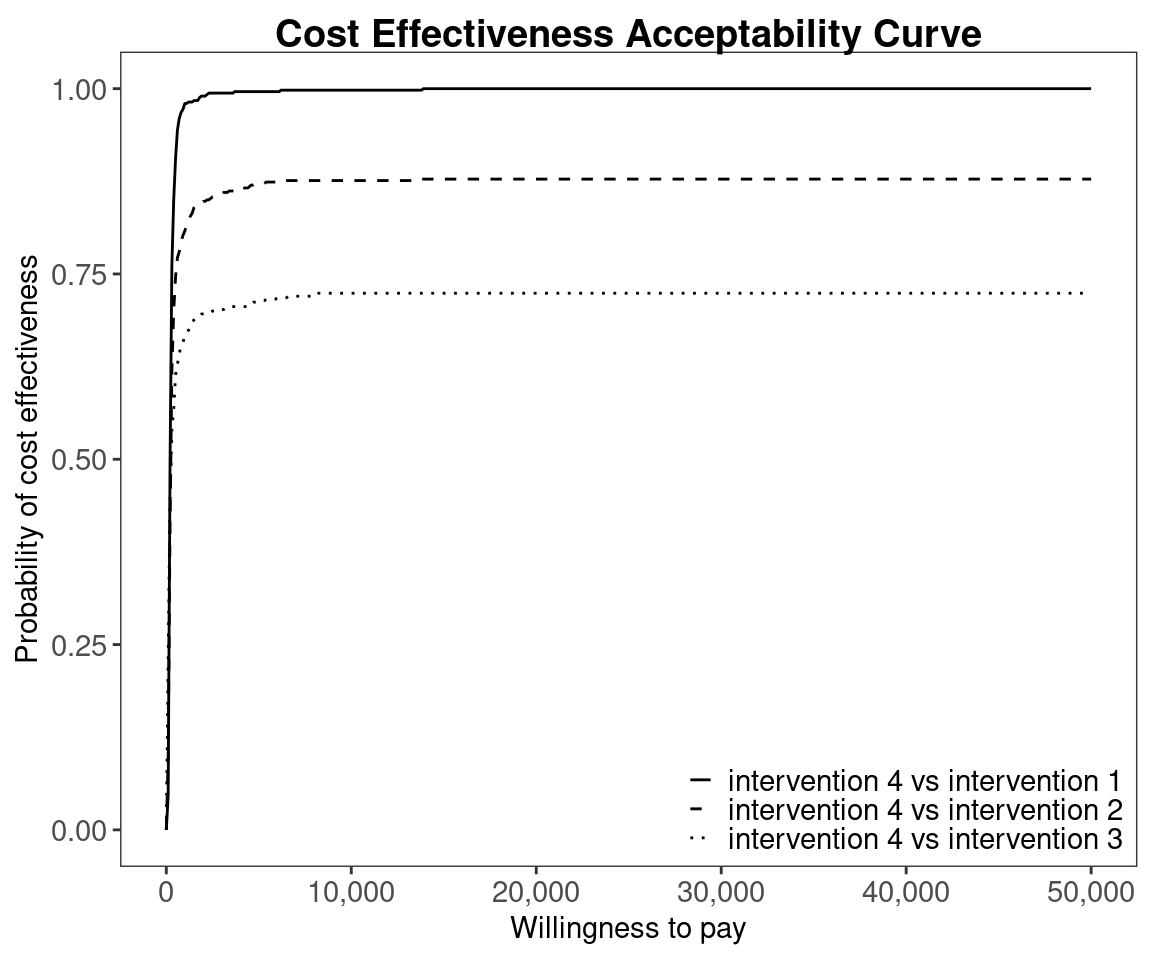ceac.plot(he, graph = "ggplot2", pos = c(1, 1))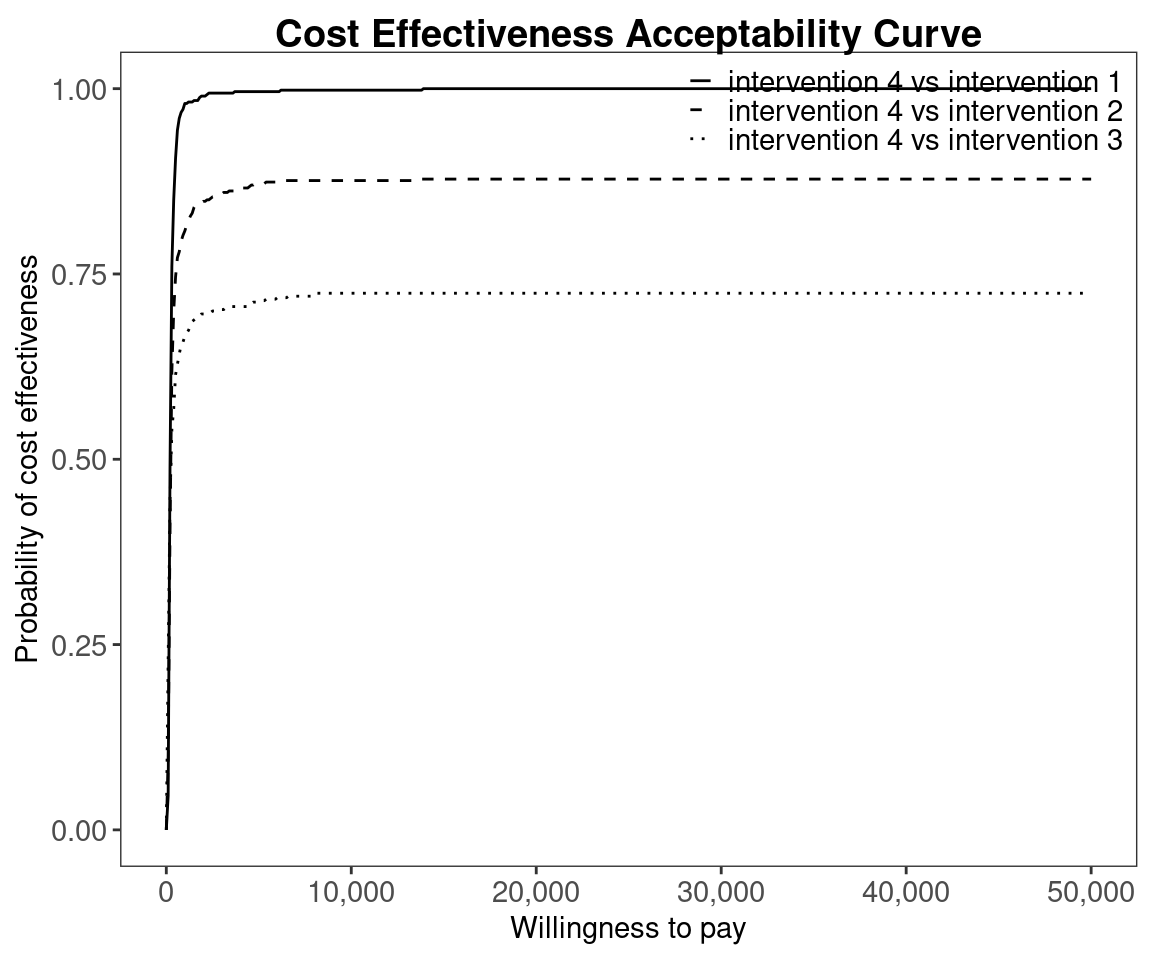Define colour palette.

mypalette <- RColorBrewer::brewer.pal(3, "Accent")

ceac.plot(he,
graph = "base",
title = "my title",
line = list(colors = mypalette),
pos = FALSE)ceac.plot(he,
graph = "ggplot2",
title = "my title",
line = list(colors = mypalette),
pos = FALSE)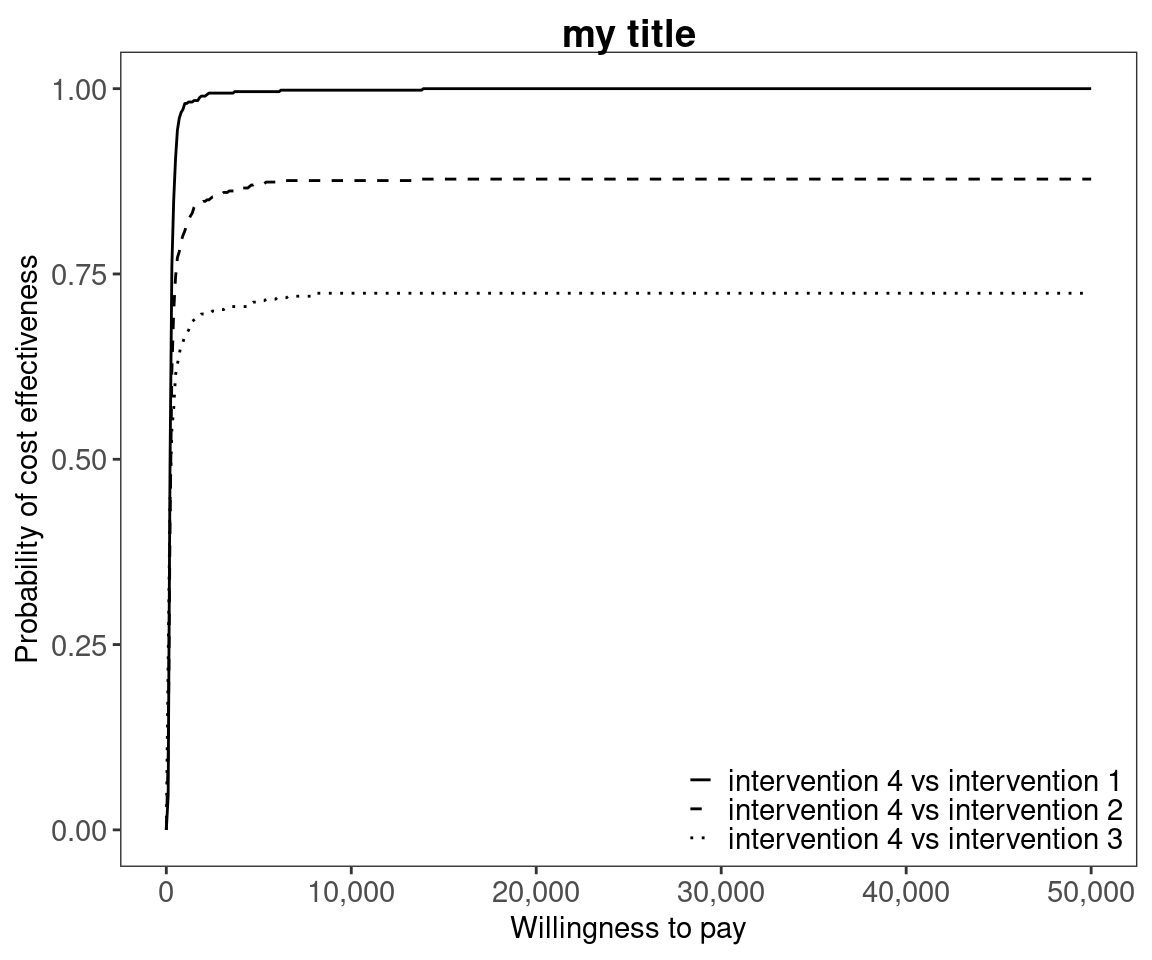Pair-wise comparisons

Again, without loss of generality, if we assume that we are interested in intervention $$i=1$$, the we wish to calculate

$p(NB_1 = \max\{NB_i : i \in S\} | k)$

This can be approximated by the following.

$\frac{1}{N} \sum_j^N \prod_{i \in S} \mathbb{I} (k \Delta e_{1,i}^j - \Delta c_{1,i}^j)$

R code

In BCEA we first we must determine all combinations of paired interventions using the multi.ce() function.

he2 <- multi.ce(he)

We can use the same plotting calls as before i.e. ceac.plot() and BCEA will deal with the pairwise situation appropriately. Note that in this case the probabilities at a given willingness to pay sum to 1.

ceac.plot(he, graph = "base")ceac.plot(he,
graph = "base",
title = "my title",
line = list(colors = "green"),
pos = FALSE)mypalette <- RColorBrewer::brewer.pal(4, "Dark2")

ceac.plot(he,
graph = "base",
title = "my title",
line = list(colors = mypalette),
pos = c(0,1))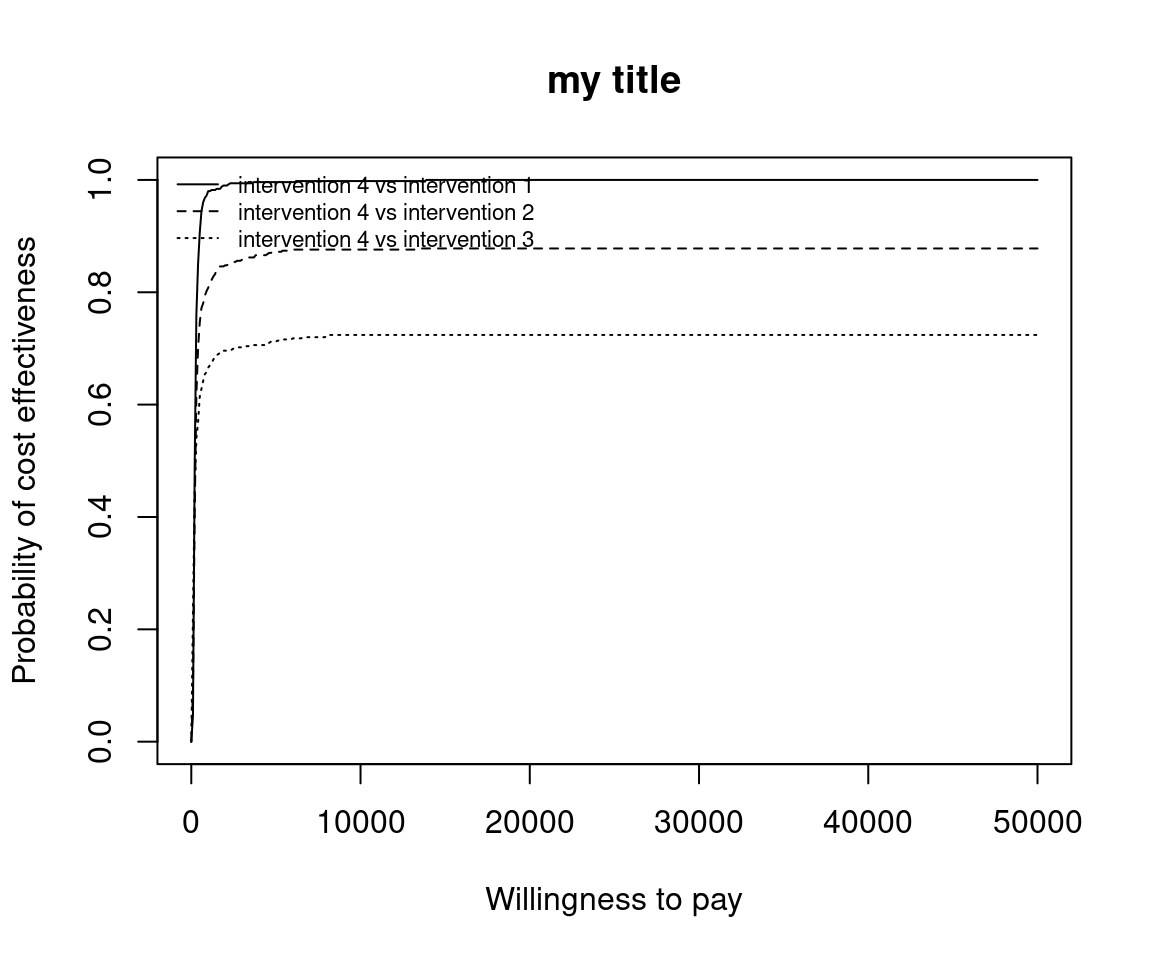ceac.plot(he,
graph = "ggplot2",
title = "my title",
line = list(colors = mypalette),
pos = c(0,1))